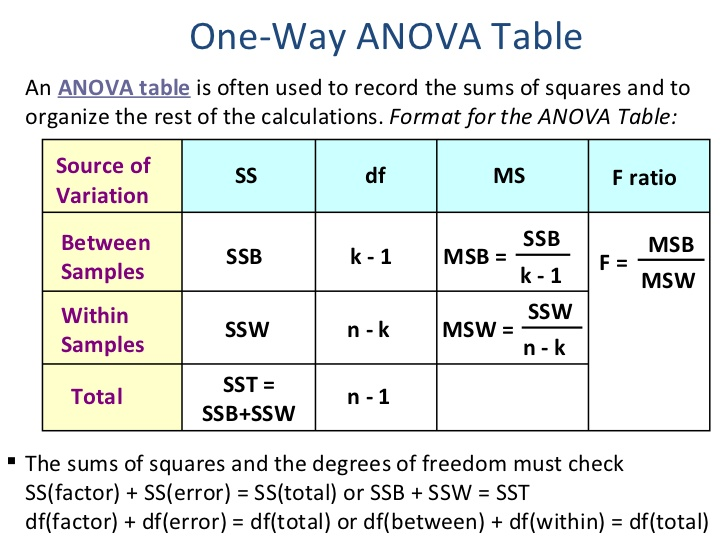SSC CGL 2020 notification states the syllabus of statistics - Study24x7Default error msg

New to Study24x7 ?

# SSC CGL 2020 notification states the syllabus of statistics (Paper3).

Updated on 15 February 2020SSC Preparation Strategies & S
Updated on 15 February 2020## SSC CGL 2020: Sampling Theory

·      Sampling is the statistical process of collecting data from small individual group

form within a large population to estimate the characteristic of whole

population.

·      Sampling theory is the field of statistics which includes gathering, analysis and

interpretation of sample data gathered from a population.

·      It uses concept of probability theory.

·      Here samples are gathered on predefined parameters and a conclusion has been

drawn after analyzing that data.

Process Of sampling:

·      Identify the population.

·      Select the sample set.

·      Define the basis of selection of sample set over the population.

·      Check thoroughly whether sample set is reflecting all the attributes of

population or not.

·      Check for the errors.

·      Define the sample set.

Types of sampling:

·      Random Sampling

·      Systematic sampling

·      Stratified sampling

SSC CGL 2020: Analysis of Variance

·      Analysis of variance is an analytical tool in statistics that splits variability of a

data set into two parts named systematic factors and random factor.

·      This test is applied in regression analysis.

·      It identifies the influence of independent variable on dependent variable.

·      This was developed by statistician Ronald Fisher.

·      It works on mean value of population set.

·      Analysis of variance tool is applied to analyze experimental data.

·      It performs multiple sample comparisons.

Formula for Analysis of variance (ANOVA):

ANOVA Coefficient =There are two types of analysis of variance possible and these are as follows:

1.  Unidirectional (one way) Method: It determines whether all the samples are same or

not.

2.  Two way Method: It is an extension of unidirectional analysis of variance.

Here we analyze the impact of two independent variables over a dependent variable.

SSC CGL 2020: Time Series Analysis

·  Time series is a sequence defined at successive equally spaced points in time

space.

·      It is a sequence of discrete time data.

·      Time series are plotted via line charts.

·     It is used in statistics, weather forecasting (height of ocean tides), earthquake

prediction, pattern recognition and signal processing.

Time Series Analysis is method for analyzing time series data to find meaningful information of data.

There are two methods available for time series analysis

1.  Time domain Method

2.  Frequency domain method

Time series pattern can be defined under following two basic components:

1.  Seasonality

2.  Trend

Identifying patterns of time series data is very important and there may be two types of pattern:

1.  Random Noise

2.  Systematic pattern

This analysis is based on assumption.

There are two basic goals of Time Series Analysis and theses are as follows:

·      Identifying the nature of event by the sequence of observed dataset.

·      Prediction of future values of variable on the basis of time series data.

Write a comment...Trending ArticlesBy CBSE CLASS 8By Edubabble LLPBy CBSE CLASS 7By CBSE CLASS 10
Related PostsServicesNeed Some Help!Connect